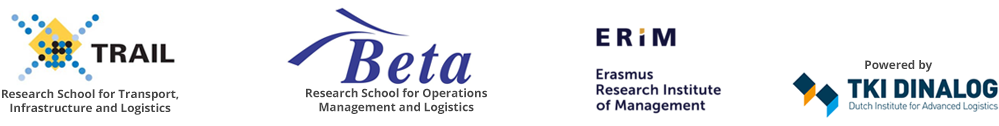# Capita selecta – Periodic Review Inventory Management and Markov Decision Processes spring 2020/21

Date:

10 & 17 March 2021

Time:

10.00 – 16.00 h

Location:

Utrecht or online – t.b.d.

Lecturer:

Prof. Eugene Feinberg (Stony Brook University, NY)

Days:

2

ECTS:

0.5 (attending only) – 2 (attending + passing the assignment)

Course fee:

Registration:

See below

Objectives:

• to rehearse the basics on inventory management
• to rehearse the basics on Markov Decision Processes
• to discuss algorithms for finding optimal policies
• to describe the structure of optimal policies including base stock models and (s,S)
• policies for problems with setup costs
• to discuss computational methods for finding optimal inventory policies
• approximations of infinite‐horizon problems with finite‐horizon problems and average-cost problems with discounted ones.

Course description:

1. Periodic review inventory control problems. Cost functions. Problems with lost sales and back orders. Lead times. Unlimited and limited storage and limited and unlimited order sizes.
2. Markov decision processes. Relations of MDPs to inventory management. Optimality criteria: finite horizon, infinite‐horizon expected discounted costs, average costs per unit time. Continuous and semi-continuous functions. Optimality equations and their properties. Algorithms for finding optimal policies. Problems with infinite state sets.
3. Optimality equations for inventory management problems. The structure of optimal policies for problems with lost sales and backorders.
4. Problems with lead times. Cash management problems. Problems with borrowing. Robust optimization of inventory management systems.

Connection with the courses “Quantitative Modeling and Analysis of Supply Chains QMASC)” and “Advanced Inventory Theory”
The former course is about inventories in general, and it focuses on multi-echelon production/inventory systems. The latter focuses on single-location models, and especially in settings with multiple items and joint replenishment costs and with multiple demand classes,

The current course focuses on the interplay between Markov Decision Processes (MDPs) and inventory control. It also demonstrates the relations of some basic facts on inventory control to simple concepts in calculus such as continuity and convexity of functions. In our days MDPs provide mathematical foundations to artificial intelligence, but for a long period of time inventory control applications were among the major factors for investigations of Markov decision processes.

Assignment:

Two sets of homework exercises (one set per day).

Program:

Lecture 1:
Periodic review inventory control problems.
Cost functions.
Problems with lost sales and back orders.
Unlimited and limited storage and limited and unlimited order sizes.

Lecture 2:
Markov decision processes (MDPs).
Relations of MDPs to inventory management.
Optimality criteria: finite horizon, infinite‐horizon expected discounted costs, average costs per unit time.
Continuous and semi-continuous functions.
MDPs with infinite state sets.
Optimality equations and their properties.
Algorithms for finding optimal policies.

Lecture 3:
Optimality equations for inventory management problems.
The structure of optimal policies for problems with lost sales and backorders; (s,S) policies.

Lecture 4:
Problems with lead times. Cash management problems. Problems with borrowing. Robust optimization of inventory management systems.

Literature:

Simchi‐Levi, Xin Chen, Julien Bramel, The logic of Logistics, Second Edition, Springer, 2005, Part II: Inventory Models

Methodology:

Course material:

E.A. Feinberg, “Optimality Conditions for Inventory Control,” Tutorials in Operations Research, INFORMS, pp. 14‐44, 2016, http://www.ams.sunysb.edu/~feinberg/public/FTUT2016.pdf

Prerequiste:

Basics in probability: discrete and continuous random variables, expectations. First course on calculus.